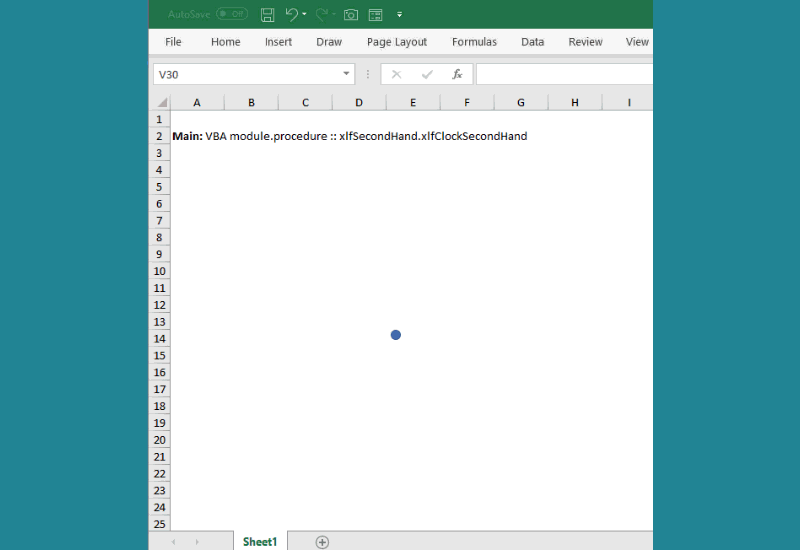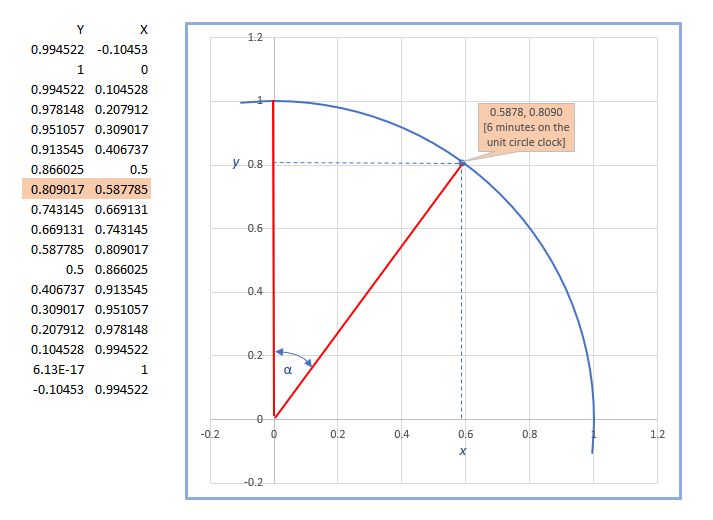# Animate a line object as analog clock hand

### [This is a project seed module]

This module provides a Quick Overview of:

1. Excel analog clocks are normally based on components of X-Y scatter charts ,  or pie charts and donut charts 
2. In this version the key element is the shape line object (figure 1)
3. VBA code is used to add the line element and provide animation for 0 to 15 minutes (code 1)
1. continuing the animation from 16 to 60
2. adding the minute and hour hands (with animation)
3. linking the animation to the computer's internal clock

## 0. Preliminary

An animated second hand of the analog clock is shown in figure 1. The logic displayed in the figure could be extended to a WS dashboard interface.Fig 1: Animated line shape - replicating the second hand of a clock in the span 0 to 15 seconds

## 1. Aspects of trigonometry and the unit circle

The logic of the clock is similar to the trigonometric aspects of the unit circle. The unit circle has a radius of 1 unit and is divided into 4 quadrants. Quadrant 1 starts at 3 on the clock and extends to 12 noon, and continues in an anti clockwise direction. In contrast, the clock in figure 1, and the circle in figure 2 start a 0, and extend in a clockwise direction. The angle of the (second) hand is shown by α. A combination of WS functions Radians, Sine, and Cos return the x and y coordinates.Fig 2: a circle with unit radius - clock face with quarter 1. The y axis is 12, and the x axis is 3

• Clock minutes to degrees; 60 minutes = 360 degrees = 2 π radians
• The angle α (6 minutes) measured in radians; = ((minutes x 6 ) / 180) x π where π = 3.14159265358979
• Using the PI function (value 3.14159265358979), 6 minutes =((6*6)/180*PI()) returns 0.628318530717959.
• Using the RADIANS function =RADIANS(6*6); returns 0.628318530717959. One minute of time is 6 degrees on the circle and 0.628319 radians
• y coordinate: cosine(α) radians =COS(0.628318530717959); returns 0.809017
• x coordinate: sine(α) radians =SIN(0.628318530717959); returns 0.587785

## 2. The VBA code

Code 1 adds the line at anchor (200, 200), constructs a coordinates array for 0 to 15 minutes, and animates the hand over the first 15 minute interval.

Code 1: Macro xlfClockSecondHand add the line, construct coordinates, and animate 0 to 15 minutes
Sub xlfClockSecondHand()
' Screen resolution: 1920 x 1080
Const ANCHORx As Double = 200
Const ANCHORy As Double = 200
Const LENGTH As Double = 100

Dim shp As Shape
Dim Leng As Double: Leng = LENGTH
Dim Second(0 To 15, 1 To 3) As Double
Dim t1 As Integer

BeginX:=ANCHORx, EndX:=ANCHORy, _
BeginY:=ANCHORy, EndY:=LENGTH)

With shp.Line
.ForeColor.RGB = RGB(255, 0, 0)
.Weight = 1
End With
VBA.DoEvents

For t1 = 0 To 15
Second(t1, 1) = WorksheetFunction.Radians(t1 * 6)
Second(t1, 2) = LENGTH * Cos(Second(t1, 1))
Second(t1, 3) = LENGTH * Sin(Second(t1, 1))
Next t1

With shp
For t1 = 1 To 15
'        Debug.Print "Top: " & .Top
'        Debug.Print "Left: " & .Left
'        Debug.Print "Width: " & .Width
'        Debug.Print "Height: " & .Height & vbNewLine
.Width = Second(t1, 3)
.Top = .Top + (Second(t1 - 1, 2) - Second(t1, 2))       ' Top + Delta y
.Height = .Height - (Second(t1 - 1, 2) - Second(t1, 2)) ' Height - Delta y
Application.Wait (Now + TimeValue("0:00:01"))
Next t1
End With
'.Flip msoFlipVertical
'.Flip msoFlipHorizontal
End Sub